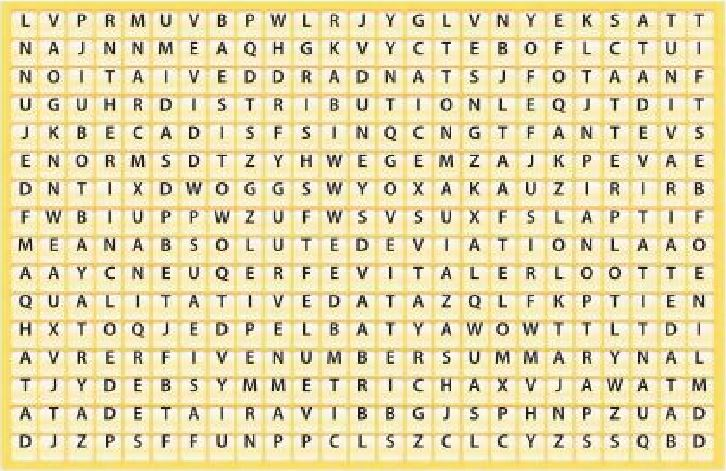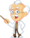Homework Explained - Math Practice 101Dear guest, you are not a registered member. As a guest, you only have read-only access to our books, tests and other practice materials.

As a registered member you can:

 Chapter Review     Vocabulary Check   Complete each sentence using the vocabulary list at the beginning of the chapter. Then circle the word that completes the sentence in the word search.Question 1 1. Data with one variable are called _____. 2. _____ is the ratio of the value of a subtotal to the value of the total. 3. Data with two variables are called _____. 4. Data that can be measured are _____. 5. The _____ uses numbers to characterize a set of data. 6. A _____ shows the relationship between data graphed as ordered pairs on the coordinate plane. 7. In a scatter plot, the _____ fit is close to most of the data points on the coordinate plane. 8. The arrangement of data values is called a _____. 9. When the left side of a distribution looks like the right side, the distribution is _____. 10. Data that can be observed or described are _____. 11. A _____ shows data that pertain to two different categories. 12. The _____ is a numerical value that shows how the data deviated from the mean. 13. The average distance between each data value and the mean is called the _____. Type below:(show solution)